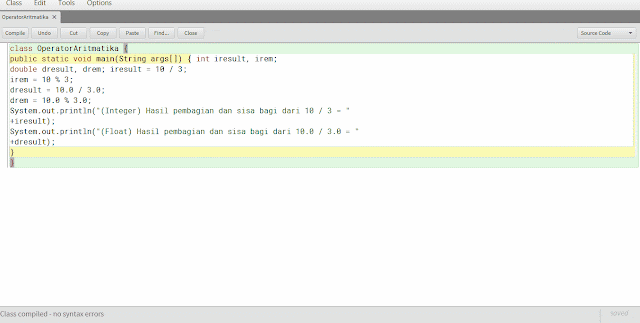Arithmetic, Logic, and Assignment Operators JAVA

Arithmetic operators

Listed below are the operators used in arithmetic calculation process for coding Java:

 Operator Information + addition -                   Subtraction (as a sign of minus) *                   Multiplication /                   Distribution % modulus ++ increment - decrement

Example Java program using arithmetic operators:
 `01` `class` `OperatorAritmatika {`
 `02` `public static void main(String args[]) { ``int` `iresult, irem;`
 `03` `double dresult, drem; iresult ``=` `10` `/` `3``;`
 `04` `irem ``=` `10` `%` `3``;`
 `05` `dresult ``=` `10.0` `/` `3.0``;`
 `06` `drem ``=` `10.0` `%` `3.0``;`
 `07` `System.out.println(``"(Integer) Hasil pembagian dan sisa bagi dari 10 / 3 = "`
 `08` `+``iresult);`
 `09` `System.out.println(``"(Float) Hasil pembagian dan sisa bagi dari 10.0 / 3.0 = "`
 `10` `+``dresult);`
 `11` `}`
 `12` `}`coding result, in the example I use with the result of integer division operator and float

Logical operators

Relational operators refers to the relationship that one can have other values, whereas the logic refers to the way in which the true and false values ​​can be linked together. Due to the relational operators produce true / false value, usually often associated with logical operators.

 Operator Information & AND | OR ^ XOR (exclusive OR) || Short-circuit OR && Short-circuit AND ! NOT

sample java program using logical operators:
 `01` `class` `operatorLogika {`
 `02` `public static void main(String args[]) { ``int` `i, j;`
 `03` `boolean b1, b2;`
 `04` `i ``=` `10``;`
 `05` `j ``=` `11``;`
 `06` `if``(i < j)`
 `07` `System.out.println(``"i < j"``); ``if``(i <``=` `j)`
 `08` `System.out.println(``"i <= j"``); ``if``(i !``=` `j)`
 `09` `System.out.println(``"i != j"``); ``if``(i ``=``=` `j)`
 `10` `System.out.println(``"this won't execute"``); ``if``(i >``=` `j)`
 `11` `System.out.println(``"this won't execute"``); ``if``(i > j)`
 `12` `System.out.println(``"this won't execute"``);`
 `13` `b1 ``=` `true; b2 ``=` `false; ``if``(b1 & b2)`
 `14` `System.out.println(``"this won't execute"``); ``if``(!(b1 & b2))`
 `15` `System.out.println(``"!(b1 & b2) is true"``); ``if``(b1 | b2)`
 `16` `System.out.println(``"b1 | b2 is true"``); ``if``(b1 ^ b2)`
 `17` `System.out.println(``"b1 ^ b2 is true"``);`
 `18` `}`
 `19` `}`coding results, will display the results of logic as an example i

Assignment operator

This operator is used to give a value to a variable or object. The symbol of this operator is "=", the form of syntax is as follows:
var = value;
No other format in the provision of value do not normally like the following example:
int x, y, z; x = y = z = 270;
other forms of shorthand assignment operator is as follows:

 Operator Example Equation + = x + = 4 x = x + 4 - = x - = 4 x = x - 4 * = x * = 4 x = x * 4 / = x / = 4 x = x / 4 % = x% = 4 x = x% 4

Berlangganan update artikel terbaru via email: